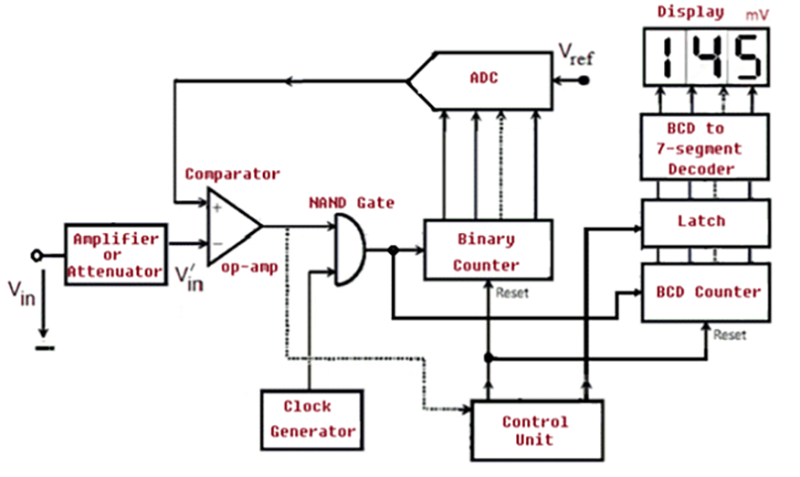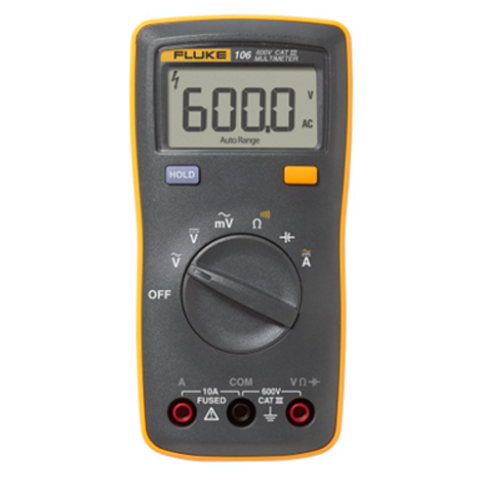Activities

# What is Digital Voltmeter

Posted byToolsWhat is Digital Voltmeter? how to choose the best one.

A digital voltmeter is a gadget that measures voltage. It measures alternating current or direct current and shows the value immediately in numeric form rather than via pointer deflection. The abbreviation DVM stands for Digital Voltmeter. Andrew Kay invented DVM in 1954.

Numerous variables influence the measuring accuracy of a digital voltmeter (DVM). These include, but are not limited to, temperature, input impedance, and fluctuation in power supply voltage. As is well known, analog instruments produce results through pointer deflection on a continuous scale. On the other hand, a digital instrument produces results in the form of discrete numerals. As a result, precision and versatility are combined.

The DVM's input range can range from 1 V to 1000 V. Precision DVM has an input resistance of 1 G or more for voltages less than 20 V.A digital voltmeter quantitatively displays the voltage values from a circuit. Initially, analog voltmeters were used to obtain voltage readings, in which a pointer or indicator moved across a scale in proportion to the circuit's voltage; later, digital voltmeters were introduced, which provide an accurate numerical display of voltage. This article will cover what a digital voltmeter is, how it works, including step-by-step functions, its various varieties, applications, and associated benefits and drawbacks.

A digital voltmeter measures the difference in electrical potential between two locations in a circuit. The voltage may be alternating current (AC) or direct current (DC). It measures the input voltage after converting it from analog to digital and displaying it in numerical format via a converter. The use of a digital voltmeter has improved the speed and precision with which readings are taken.

How does Digital Voltmeter work?

A Digital Voltmeter's operation can be classified into five functional divisions. They are as follows:

• Pulse Generator
• Voltage Control and Gating
• Counting Clock Pulses
• Analog to Digital Conversion
• Latching and Display Section

Block diagram of Digital VoltmeterFig. 2 – Diagram of the Basic Blocks of a Digital Voltmeter

Types of Digital Voltmeter

Digital Voltmeter is broadly classified into four types. They are:

• Ramp Type Digital Voltmeter
• Integrating Digital Voltmeter
• Continuous Balance Digital Voltmeter
• Successive approximation Digital Voltmeter

Applications of Digital Voltmeter

• A digital voltmeter is used to determine the true voltage of various components.
• The DVM is frequently used to determine whether there is power in a circuit, such as a mains outlet.
• Knowing the voltage across a circuit, current can be calculated

Fluke 106 Palm-sized Digital MultimeterKey Features

• It can measure voltage, resistance, Continuity as

well as capacitance

• Input terminal for ac and dc current measurements to

10 A and current

• It can hold Data

• The output is shown digitally, which avoids human reading errors.
• In comparison to analog meters, readings are precise and rapid.
• In comparison to analog voltmeters, DVMs provide more accuracy and versatility.
• The output of DVM can be fed to memory devices for further computations.
• The decreased size of DVM increases the portability of the instrument.
• DVM offers an accuracy of 0.5% + 1 digit and the operating temperature range is -5 ⁰C to

55 ⁰C.

• Digital Voltmeter is more stable and reliable.
• Smaller in size and cost-effective.
• AC and DC voltages can measure.
• The latest generation of DVMs incorporates microcontrollers that store the readings for later processing.
• DVM is void of Parallax errors.
• DVMs have automatic range selection.
• DVMs have high input impedance.
• DVM provides numerical readouts that eliminate observational errors. Thus providing